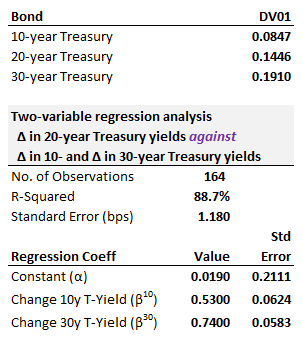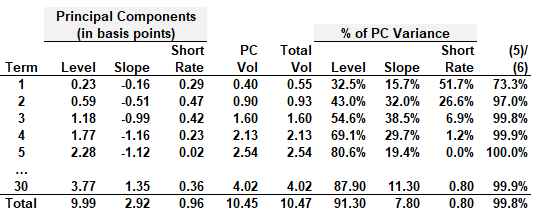# P2.T5.22.15. Fixed income regression and principal component analysis (PCA)

#### Nicole Seaman

##### Director of FRM Operations
Staff member
Subscriber
Learning objectives: Calculate the face value of multiple offsetting swap positions needed to carry out a two-variable regression hedge. Compare and contrast level and change regressions. Describe principal component analysis and explain how it is applied to constructing a hedging portfolio.

Questions:

22.15.1. Consider the following regression of daily yield changes of the 20-year Treasury bond against daily yield changes in 10-year and 30-year bonds (similar to Tuckman's two-variable regression hedge):A relative value trader perceives that the 20-year yield is too high (aka, price is too low) relative to the 10- and 30-year bonds. She executives a butterfly trade to exploit her view: she buys \$100.0 million face amount of the 20-year Treasury bond and sells both the 10- and 30-year bonds.

How does the dollar value of '01 (i.e., DV01) of her hedging position compare to the DV01 of the 20-year bond (aka position being hedged)?

a. 41.% less
b. 13% less
c. +12% more
d. +27% more

22.15.2. Sam wants to regress bond Y against bond X, but he cannot decide between a level or change regression

• Regress yield level of Y(t) on yield level of X(t); aka, level-on-level regression
• Regress yield change, ΔY(t), on yield change of ΔY(t); aka, change-on-change regression
Currently, the yield of bond X is constant at 5.0%, while the yield of bond Y was 1.0% yesterday. The most likely go-forward scenario is that the yield of bond Y will trend from its current value of 1.0% to approximately its "model value" of 5.0%.

Which regression is most appropriate in this circumstance?

a. Any change-on-change regression
b. Change-on-change regression but employing least-squares estimates that are BLUE
c. Level-on-level regression with autoregressive, AR(1), error dynamics, ε(t) = ρ*ε(t-1) + ν(t)
d. Level-on-level regression with coefficients reversed (aka, reverse regression) to smooth decay and enforce lower bound

22.15.3. The table below replicates (but the seven middle rows are truncated: 6 to 10, 15, and 20-year terms) Tuckman's principal component analysis (PCA) of USD Libor swap rates from June 1, 2020, to July 16, 2021. Source: Tuckman's Fixed Income Securities, 5th edition.The dataset consists of 13 interest rate factors. About this principal component analysis (PCA), each of the following statements is TRUE except which is false?

a. While the interest rate factor covariance matrix contains 78 covariances (plus 13 variances), the principal component covariance matrix is a diagonal matrix
b. A fixed income PCA is convenient because it enables the user to map term structure volatility to the desired fundamental factors that contribute to an easily explainable narrative; in particular, level (aka, parallel shift), slope, and twist
c. The PCA constructs 13 principal components (PC), but conveniently only a limited number of PCs are needed, in this case, three, to sufficiently characterize term structure fluctuations
d. The PC volatility ("PC vol") of the one-year rate is sqrt[0.23^2 + (-0.16)^2 + 0.29^2] because the PCs are orthogonal by construction